Skip to main content Accessibility help
Home
Hostname: page-component-747cfc64b6-xl4lj Total loading time: 0.252 Render date: 2021-06-13T12:30:33.635Z Has data issue: true Feature Flags: { "shouldUseShareProductTool": true, "shouldUseHypothesis": true, "isUnsiloEnabled": true, "metricsAbstractViews": false, "figures": true, "newCiteModal": false, "newCitedByModal": true, "newEcommerce": true }Compositio Mathematica

# Decomposition of degenerate Gromov–Witten invariants

Published online by Cambridge University Press:  19 November 2020

Corresponding
E-mail address:

## Abstract

We prove a decomposition formula of logarithmic Gromov–Witten invariants in a degeneration setting. A one-parameter log smooth family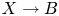$X \longrightarrow B$ with singular fibre over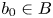$b_0\in B$ yields a family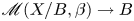$\mathscr {M}(X/B,\beta ) \longrightarrow B$ of moduli stacks of stable logarithmic maps. We give a virtual decomposition of the fibre of this family over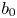$b_0$ in terms of rigid tropical maps to the tropicalization of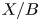$X/B$. This generalizes one aspect of known results in the case that the fibre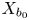$X_{b_0}$ is a normal crossings union of two divisors. We exhibit our formulas in explicit examples.

## MSC classification

Type
Research Article
Information
Compositio Mathematica , October 2020 , pp. 2020 - 2075
Copyright
© The Author(s) 2020

## Access options

Get access to the full version of this content by using one of the access options below.

## Footnotes

Research by D.A. was supported in part by NSF grants DMS-1162367, DMS-1500525 and DMS-1759514. Research by Q.C. was supported in part by NSF grant DMS-1403271 and DMS-1560830. M.G. was supported by NSF grant DMS-1262531, EPSRC grant EP/N03189X/1 and a Royal Society Wolfson Research Merit Award. Research by B.S. was partially supported by NSF grant DMS-1903437.

## References

Abramovich, D., Caporaso, L. and Payne, S., The tropicalization of the moduli space of curves, Ann. Sci. Éc. Norm. Supér. (4) 48 (2015), 765809.CrossRefGoogle Scholar
Abramovich, D. and Chen, Q., Stable logarithmic maps to Deligne-Faltings pairs II, Asian J. Math. 18 (2014), 465488.CrossRefGoogle Scholar
Abramovich, D., Chen, Q., Gross, M. and Siebert, B., Punctured logarithmic maps, Preprint (2020), arXiv:2009.07720.Google Scholar
Abramovich, D., Chen, Q., Marcus, S. and Wise, J., Boundedness of the space of stable logarithmic maps, J. Eur. Math. Soc. (JEMS) 19 (2017), 27832809.CrossRefGoogle Scholar
Abramovich, D. and Karu, K., Weak semistable reduction in characteristic 0, Invent. Math. 139 (2000), 241273.CrossRefGoogle Scholar
Abramovich, D. and Wise, J., Birational invariance in logarithmic Gromov–Witten theory, Compos. Math. 154 (2018), 595620.CrossRefGoogle Scholar
Arbarello, E., Cornalba, M. and Griffiths, P., Geometry of algebraic curves, Volume II (Springer, 2011).CrossRefGoogle Scholar
Behrend, K., Gromov–Witten invariants in algebraic geometry, Invent. Math. 127 (1997), 601617.CrossRefGoogle Scholar
Behrend, K. and Fantechi, B., The intrinsic normal cone, Invent. Math. 128 (1997), 4588.CrossRefGoogle Scholar
Behrend, K. and Manin, Y., Stacks of stable maps and Gromov–Witten invariants, Duke Math. J. 85 (1996), 160.CrossRefGoogle Scholar
Bryan, J. and Leung, N. C., The enumerative geometry of$K3$ surfaces and modular forms, J. Amer. Math. Soc. 13 (2000), 371410.CrossRefGoogle Scholar
Burgos Gil, J. and Sombra, M., When do the recession cones of a polyhedral complex form a fan? Discrete Comput. Geom. 46 (2011), 789798.CrossRefGoogle Scholar
Cadman, C., Using stacks to impose tangency conditions on curves, Amer. J. Math. 129 (2007), 405427.CrossRefGoogle Scholar
Cavalieri, R., Chan, M., Ulirsch, M. and Wise, J., A moduli stack of tropical curves, Forum Math. Sigma 8 (2020), e23.Google Scholar
Chen, Q., Stable logarithmic maps to Deligne–Faltings pairs I, Ann. of Math. (2) 180 (2014), 455521.CrossRefGoogle Scholar
Costello, K., Higher genus Gromov–Witten invariants as genus zero invariants of symmetric products, Ann. of Math. (2) 164 (2006), 561601.CrossRefGoogle Scholar
Gross, M. and Siebert, B., Logarithmic Gromov–Witten invariants, J. Amer. Math. Soc. 26 (2013), 451510.CrossRefGoogle Scholar
Gross, M. and Siebert, B., Intrinsic mirror symmetry, Preprint (2019), arXiv:1909.07649.Google Scholar
Kato, K., Logarithmic structures of Fontaine–Illusie, in Algebraic analysis, geometry, and number theory, Baltimore, MD, 1988 (Johns Hopkins University Press, 1989), 191–224.Google Scholar
Kato, F., Exactness, integrality, and log modifications, Preprint (1999), arXiv:math/9907124.Google Scholar
Kato, F., Log smooth deformation and moduli of log smooth curves, Internat. J. Math. 11 (2000), 215232.CrossRefGoogle Scholar
Kempf, G., Knudsen, F., Mumford, D. and Saint-Donat, B., Toroidal embeddings I, Lecture Notes in Mathematics, vol. 339 (Springer, 1973).CrossRefGoogle Scholar
Kim, B., Lho, H. and Ruddat, H., The degeneration formula for stable log maps, Preprint (2018), arXiv:1803.04210.Google Scholar
Knudsen, F., The projectivity of the moduli space of stable curves. II. The stacks$M_{g,n}$, Math. Scand. 52 (1983), 161199.CrossRefGoogle Scholar
Kresch, A., Cycle groups for Artin stacks, Invent. Math. 138 (1999), 495536.CrossRefGoogle Scholar
Li, J., A degeneration formula of GW-invariants, J. Differential Geom. 60 (2002), 199293.CrossRefGoogle Scholar
Mandel, T. and Ruddat, H., Descendant log Gromov–Witten invariants for toric varieties and tropical curves, Trans. Amer. Math. Soc. 373 (2020), 11091152.CrossRefGoogle Scholar
Manolache, C., Virtual pull-backs, J. Algebraic Geom. 21 (2012), 201245.CrossRefGoogle Scholar
Mikhalkin, G., Enumerative tropical algebraic geometry in$\mathbb {R}^2$, J. Amer. Math. Soc. 18 (2005), 313377.CrossRefGoogle Scholar
Mochizuki, S., The geometry of the compactification of the Hurwitz scheme, Publ. Res. Inst. Math. Sci. 31 (1995), 355441.CrossRefGoogle Scholar
Nishinou, T. and Siebert, B., Toric degenerations of toric varieties and tropical curves, Duke Math. J. 135 (2006), 151.CrossRefGoogle Scholar
Ogus, A., Lectures on logarithmic algebraic geometry (Cambridge University Press, 2018).CrossRefGoogle Scholar
Olsson, M., Logarithmic geometry and algebraic stacks, Ann. Sci. École Norm. Sup. (4) 36 (2003), 747791.CrossRefGoogle Scholar
Parker, B., Holomorphic curves in exploded manifolds: compactness, Adv. Math. 283 (2015), 377457.CrossRefGoogle Scholar
Parker, B., Tropical gluing formulae for Gromov–Witten invariants, Preprint (2017), arXiv:1703.05433.Google Scholar
Parker, B., Holomorphic curves in exploded manifolds: regularity, Geom. Topol. 23 (2019), 16211690.CrossRefGoogle Scholar
Parker, B., Holomorphic curves in exploded manifolds: Kuranishi structure, Preprint (2019), arXiv:1301.4748.Google Scholar
Parker, B., Holomorphic curves in exploded manifolds: virtual fundamental class, Geom. Topol. 23 (2019), 18771960.CrossRefGoogle Scholar
Ranganathan, D., Logarithmic Gromov–Witten theory with expansions, Preprint (2020), arXiv:1903.09006.Google Scholar
The Stacks Project Authors, Stacks Project, http://stacks.math.columbia.edu (2017).Google Scholar
Ulirsch, M., Tropical geometry of logarithmic schemes, PhD thesis, Brown University (2015).Google Scholar
Ulirsch, M., Functorial tropicalization of logarithmic schemes: the case of constant coefficients, Proc. Lond. Math. Soc. (3) 114 (2017), 10811113.Google Scholar
Ulirsch, M., A non-Archimedean analogue of Teichmüller space and its tropicalization, Preprint (2020), arXiv:2004.07508.Google Scholar
Wise, J., Moduli of morphisms of logarithmic schemes, Algebra Number Theory 10 (2016), 695735.CrossRefGoogle Scholar
Wise, J., Uniqueness of minimal morphisms of logarithmic schemes, Algebr. Geom. 6 (2019), 5063.CrossRefGoogle Scholar
Yu, T. Y., Enumeration of holomorphic cylinders in log Calabi-Yau surfaces. II. Positivity, integrality and the gluing formula, Geom. Topol., to appear. Preprint (2020), arXiv:1608.07651.Google Scholar
2
Cited by

# Send article to Kindle

To send this article to your Kindle, first ensure no-reply@cambridge.org is added to your Approved Personal Document E-mail List under your Personal Document Settings on the Manage Your Content and Devices page of your Amazon account. Then enter the ‘name’ part of your Kindle email address below. Find out more about sending to your Kindle. Find out more about sending to your Kindle.

Note you can select to send to either the @free.kindle.com or @kindle.com variations. ‘@free.kindle.com’ emails are free but can only be sent to your device when it is connected to wi-fi. ‘@kindle.com’ emails can be delivered even when you are not connected to wi-fi, but note that service fees apply.

Find out more about the Kindle Personal Document Service.

Decomposition of degenerate Gromov–Witten invariants
Available formats
×

# Send article to Dropbox

To send this article to your Dropbox account, please select one or more formats and confirm that you agree to abide by our usage policies. If this is the first time you use this feature, you will be asked to authorise Cambridge Core to connect with your <service> account. Find out more about sending content to Dropbox.

Decomposition of degenerate Gromov–Witten invariants
Available formats
×

# Send article to Google Drive

To send this article to your Google Drive account, please select one or more formats and confirm that you agree to abide by our usage policies. If this is the first time you use this feature, you will be asked to authorise Cambridge Core to connect with your <service> account. Find out more about sending content to Google Drive.

Decomposition of degenerate Gromov–Witten invariants
Available formats
×
×

#### Reply to:Submit a response

Please enter your response.

#### Your details

Please enter a valid email address.

#### Conflicting interests

Do you have any conflicting interests? *